# The acceleration due to gravity of a particle falling toward the earth is a = —gR2/r2,where ris the distance from the centerof the earth to the particle, Ris the radius of the earth, and g is the acceleration due to gravity at the surface of the earth. if R= 3960 mi, calculate the escape velocity,that is. the minimum velocity with which a particle must be projected vertically upw ard from the surface of the earth if it is not to return to the earth, (Hint: τ =0 for r = x.)

Question-AnswerCategory: Engineering MechanicsThe acceleration due to gravity of a particle falling toward the earth is a = —gR2/r2,where ris the distance from the centerof the earth to the particle, Ris the radius of the earth, and g is the acceleration due to gravity at the surface of the earth. if R= 3960 mi, calculate the escape velocity,that is. the minimum velocity with which a particle must be projected vertically upw ard from the surface of the earth if it is not to return to the earth, (Hint: τ =0 for r = x.)

The acceleration due to gravity of a particle falling toward the earth is a = —gR2/r2,where ris the distance from the centerof the earth to the particle, Ris the radius of the earth, and g is the acceleration due to gravity at the surface of the earth. if  R= 3960 mi, calculate the escape velocity,that is. the minimum velocity with which a particle must be projected vertically upw ard from the surface of the earth if it is not to return to the earth, (Hint: τ =0 for r = x.)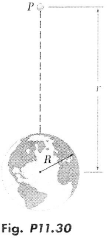Step: 1

Draw the schematic diagram of the particle and the earth.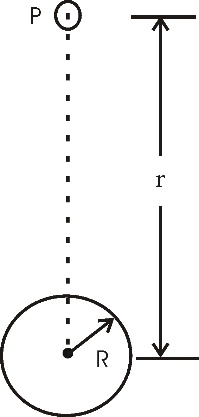Step: 2

The acceleration due to gravity of a particle falling toward the earth is,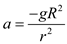Here, v is the escape velocity, g is the acceleration due to gravity, R is the radius of the earth, and r is the distance from the centre of the earth to the particle.
Apply Integration on both sides,…… (1)
Substitute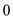for v andfor r.Step: 3

Consider the equation (1).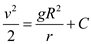Substitute 0 for C.…… (2)

Step: 4

To calculate the minimum velocity at the surface of earth. That is, when,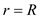.
Substitute R for r.Substitutefor R andfor g.Therefore, the escape velocity is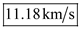.